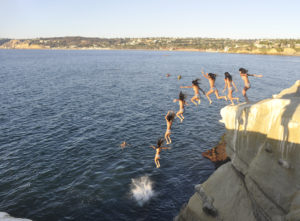## Why It Matters: Quadratic Equations and Complex Numbers

In algebra, a quadratic equation (from the Latin quadratus for “square”) is any equation having the form

$ax^2+bx+c=0$

where x represents an unknown, and a, b, and c represent known numbers such that a is not equal to  $0$. If a =$0$, then the equation is linear, not quadratic. The numbers a, b, and c are the coefficients of the equation and may be distinguished by calling them, respectively, the quadratic coefficient, the linear coefficient, and the constant or free term.

Joan and her friend Hazel have decided to go cliff jumping at their favorite spot along the river. Hazel is an amateur photographer who just got a new camera that has a continuous shooting mode. Hazel takes a picture of Joan jumping off a cliff into the river that looks like this:Cliff jumping off a rock into the ocean. The trajectory of the cliff jumper is parabolic.

When she sees the picture, Joan is reminded of what she learned in her math class about the shape of graphs of quadratic functions. She tells Hazel that the path of her jump reminds her of an upside down parabola. “Oh yeah!” responds Hazel, “I remember that from high school physics. We learned how to calculate how long it takes something to fall due to gravity.”

Joan wonders if she could calculate how long she was in the air when she made the jump off the cliff, so when they are done playing in the river, they go back to Hazels’ house to see if she still has her old physics notes.

In her notes, Hazel finds the following equation:

$h(t)=-10\frac{m}{s^2}t^2+v_{o}t+h_{o}$

She had labeled the equation with arrows in the following way: $-10\frac{m}{s^2}$ is gravity, $v_{o}$ is the initial speed of the object, and $h_{o}$ is the initial height of the object.

Hazel and Joan think they can figure out how long they were in the air as they jumped from the cliff into the river. Stay tuned for the end of the module when we see how they do it.

## Contribute!

Did you have an idea for improving this content? We’d love your input.